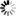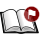Normal view

# Cosmic evolution of black holes and the M-sigma relation a thesis submitted for the degree of doctor of philosophy in the department of physics, Pondicherry University Dipanweeta Bhattacharyya [Ph.D Thesis]

Material type:TextPublication details: Bangalore Indian Institute of Astrophysics 2020Description: xvi, 195pSubject(s): Online resources: Dissertation note: Doctor of Philosophy Pondicherry University, Puducherry 2020 Summary: The connection of the SMBHs to their host galaxies is evidenced by the strong correlation between the mass of SMBH and velocity dispersion, σ, of the stars in rest of the galaxy. This is somewhat surprising because the velocity dispersion is measured for the stars which are too far from the SMBH to be a ected by its gravitational eld. Its origin is still a topic of debate. This relation is important since the mass of SMBH which is very di cult to measure directly can be calculated with relatively better precision using a quantity σ (the velocity dispersion of stars far from the SMBH) which is easier to measure for nearby systems. The cosmological M• − σ relation is given by the equation M•(z) = k0(z)σ p(z) , (1) We worked on the static as well as the dynamical aspects of this relation. For the static aspect, we deduce the M• − σ relation for elliptical (spherical) galaxies by calculating σ from their observed intensity pro les and for the dynamical aspect, we compute the evolution of this relation as an application of our model of the evolution of mass and spin of the black hole. To investigate the M• − σ relation, we consider realistic elliptical (spherical) galaxy pro les that are taken to follow a single power law density pro le given by ρ(r) = ρ0(r/r0) −γ or the Nuker intensity pro le. We calculate the density using Abel's formula in the latter case by employing the derived stellar potential in both cases, we derive the distribution function f(E) of the stars in presence of the supermassive black hole (SMBH) at the center and hence compute the line of sight (LOS) velocity dispersion as a function of radius. For the typical range of values for masses of SMBH, we obtain M• ∝ σ p for di erent pro les. An analytical relation p = (2γ + 6)/(2 + γ) is found which is in reasonable agreement with observations (for γ = 0.75 - 1.4, p = 3.6 - 5.3). Assuming that a proportionality relation holds between the black hole mass and bulge mass, M• = fbMb, and applying this to several galaxies we nd the individual best t values of p as a function of f; also by minimizing χ 2 , we nd the best t global p and fb. For Nuker pro les we nd that p = 3.81 ± 0.004 and fb = (1.23 ± 0.09) × 10−3 which are consistent with the observed ranges. We build an evolution model of the central black hole that is mainly dependent on the processes of gas accretion, the capture of stars, mergers as well as electromagnetic torque. In the case of gas accretion in the presence of cooling sources, the ow is momentum-driven, after which the black hole reaches a saturated mass and subsequently, it grows only by stellar capture and mergers. We model the evolution of the black hole mass and spin with the initial seed mass and spin as a function of redshift in a ΛCDM cosmology. For the stellar capture, we have assumed a powerlaw density pro le for the stellar cusp in a framework of relativistic loss cone theory that includes the e ect of the black hole spin, Carter's constant, loss cone angular momentum, and capture radius. The predicted capture rates of 10−5 − 10−6 yr−1 are closer to the observed range. We have considered the merger activity to be e ective for z . 4, and we self-consistently include the Blandford-Znajek torque for spin evolution. We predict the impact of the evolution on the M• − σ relation and show that our results are consistent with available observations. We model the speci c cases of the quasars ULASJ134208.10+092838.61 (z=7.54), ULASJ112001.48+064124.3 (z=7.08) and DELSJ003836.10-152723.6 (z=7.02) and retrodict their formation parameters at z = {10, 15, 20} to nd that heavy seeds of 107M are required. Our model is useful for building demographics of the black holes, in constraining formation scenarios and in providing inputs for future simulations. We present some preliminary results of the derivation of the M• − σ relation in axisymmetric systems and a formulation of initial seed mass and spin functions of black holes. We also discuss future work stemming from the ideas in this Thesis.
Tags from this library: No tags from this library for this title.
Star ratingsAverage rating: 0.0 (0 votes)
Holdings
Item type Current library Shelving location Call number Status Date due BarcodeThesis & Dissertations IIA Library-Bangalore General Stacks Available 20436

Ph.D. Thesis, Pondicherry University, Puducherry

Doctor of Philosophy Pondicherry University, Puducherry 2020

The connection of the SMBHs to their host galaxies is evidenced by the strong correlation between the mass of SMBH and velocity dispersion, σ, of the stars in rest of
the galaxy. This is somewhat surprising because the velocity dispersion is measured
for the stars which are too far from the SMBH to be a ected by its gravitational eld.
Its origin is still a topic of debate. This relation is important since the mass of SMBH
which is very di cult to measure directly can be calculated with relatively better precision using a quantity σ (the velocity dispersion of stars far from the SMBH) which
is easier to measure for nearby systems. The cosmological M• − σ relation is given by
the equation
M•(z) = k0(z)σ
p(z)
, (1)
We worked on the static as well as the dynamical aspects of this relation. For the static
aspect, we deduce the M• − σ relation for elliptical (spherical) galaxies by calculating
σ from their observed intensity pro les and for the dynamical aspect, we compute the
evolution of this relation as an application of our model of the evolution of mass and
spin of the black hole.
To investigate the M• − σ relation, we consider realistic elliptical (spherical) galaxy
pro les that are taken to follow a single power law density pro le given by ρ(r) =
ρ0(r/r0)
−γ or the Nuker intensity pro le. We calculate the density using Abel's formula
in the latter case by employing the derived stellar potential in both cases, we derive
the distribution function f(E) of the stars in presence of the supermassive black hole
(SMBH) at the center and hence compute the line of sight (LOS) velocity dispersion
as a function of radius. For the typical range of values for masses of SMBH, we obtain
M• ∝ σ
p
for di erent pro les. An analytical relation p = (2γ + 6)/(2 + γ) is found
which is in reasonable agreement with observations (for γ = 0.75 - 1.4, p = 3.6 - 5.3).
Assuming that a proportionality relation holds between the black hole mass and bulge
mass, M• = fbMb, and applying this to several galaxies we nd the individual best t
values of p as a function of f; also by minimizing χ
2
, we nd the best t global p and
fb. For Nuker pro les we nd that p = 3.81 ± 0.004 and fb = (1.23 ± 0.09) × 10−3
which are consistent with the observed ranges.
We build an evolution model of the central black hole that is mainly dependent on
the processes of gas accretion, the capture of stars, mergers as well as electromagnetic torque. In the case of gas accretion in the presence of cooling sources, the
ow is momentum-driven, after which the black hole reaches a saturated mass and
subsequently, it grows only by stellar capture and mergers. We model the evolution
of the black hole mass and spin with the initial seed mass and spin as a function of
redshift in a ΛCDM cosmology. For the stellar capture, we have assumed a powerlaw density pro le for the stellar cusp in a framework of relativistic loss cone theory
that includes the e ect of the black hole spin, Carter's constant, loss cone angular
momentum, and capture radius. The predicted capture rates of 10−5 − 10−6
yr−1
are
closer to the observed range. We have considered the merger activity to be e ective
for z . 4, and we self-consistently include the Blandford-Znajek torque for spin evolution. We predict the impact of the evolution on the M• − σ relation and show that
our results are consistent with available observations. We model the speci c cases
of the quasars ULASJ134208.10+092838.61 (z=7.54), ULASJ112001.48+064124.3
(z=7.08) and DELSJ003836.10-152723.6 (z=7.02) and retrodict their formation parameters at z = {10, 15, 20} to nd that heavy seeds of 107M are required. Our
model is useful for building demographics of the black holes, in constraining formation
scenarios and in providing inputs for future simulations.
We present some preliminary results of the derivation of the M• − σ relation in axisymmetric systems and a formulation of initial seed mass and spin functions of black
holes. We also discuss future work stemming from the ideas in this Thesis.

There are no comments on this title.